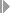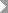Show TOC

###(04) Quantity-Based Method#### Use

You can use the quantity-based POC method for:

• Sales orders

• Projects

• Internal orders with revenue, service orders with revenue, maintenance orders with revenue

With quantity-based results analysis, you can calculate the costs affecting net income on the basis of an actual quantity. You use the Base Quantity field in Customizing for the valuation method to control which quantities are used in the calculation. With sales orders, you can use the billing quantity or the delivery quantity as a basis for the actual quantity. The planned quantity then equals the quantity in the sales order item.Note

If you have calculated the planned costs in a sales order cost estimate, the order quantity is the lot size of the cost estimate. If you have calculated the planned costs in the standard cost estimate for the material, the costs are converted to the order quantity. This also converts the costs that are independent of the lot size.

End of the note.

#### Prerequisites

You have planned costs and quantities.

Choose a results analysis method in simplified Customizing for Product Cost by Sales Order underPeriod-End ClosingResults AnalysisValuation Method.

#### Features

POC = Q(a) / Q(p)

C(PA) = POC * C(p) = Q(a) / Q(p) * C(p)

R(PA) = R(a)

Revenue affecting net income equals actual revenue. Costs affecting net income are the planned costs multiplied by the quotient of actual quantity and planned quantity. Costs affecting net income can be allocated to CO-PA together with revenue affecting net income.

If actual costs are greater than the costs affecting net income, the system creates capitalized costs.

If actual costs are less than the costs affecting net income, the system creates reserves for unrealized costs.

Inventory values and reserves for unrealized costs can be transferred to FI and EC-PCA when you settle.

#### Example

You have planned revenues of USD 200,000 and costs of USD 120,000 for your sales order. The order quantity is 100 units. The base quantity is the invoiced quantity.

Period 01

In period 01 you have actual costs of USD 20,000. You have not billed the customer. In results analysis, the system calculates the following data:

• No costs affecting net income

• No revenue affecting net income

• Capitalized costs of USD 20,000 using the formula: Capitalized Costs = Actual Costs – Costs Affecting Net Income

You then settle the capitalized costs to FI and EC-PCA.

The following values are reported in CO-PA:

Profitability Analysis

 Revenue 0 Cost of sales 0 Profit 0

The income statement shows the following values:

Income Statement

 Expense Revenue Actual costs 20,000 Capitalized costs 20,000 20,000 20,000

Period 02

In period 02 actual costs increase to USD 80,000. You deliver to your customer and send him a milestone invoice for USD 100,000. The invoiced quantity is 40 units. The order is partially delivered and partially billed. In results analysis, the system calculates the following data:

• Revenue affecting net income of USD 100,000

• Costs affecting net income in the amount of USD 48,000 using the formula: Costs Affecting Net Income = Actual Quantity / Planned Quantity * Planned Costs

• Capitalized costs of USD 32,000

You then settle the following:

• Revenue affecting net income to CO-PA

• Costs affecting net income to CO-PA

• The change in the capitalized costs to FI and EC-PCA

The following values are reported in CO-PA:

Profitability Analysis

 Revenue 100,000 Cost of sales 48,000 Profit 52,000

The income statement shows the following values:

Income Statement

 Expense Revenue Actual costs 80,000 Actual revenue 100,000 Profit 52,000 Capitalized costs 32,000 132,000 132,000

Period 03

In period 03 actual costs increase to USD 90,000. You deliver a second amount to your customer and send him a second milestone billing for USD 90,000. Total revenue is USD 190,000. The total invoiced quantity is 80 units. The order is partially delivered and partially billed. In results analysis, the system calculates the following data:

• Costs affecting net income of USD 96,000

• Revenue affecting net income of USD 190,000

• Reserves for unrealized costs of USD 6,000

• No capitalized costs The capitalized costs calculated in the prior period are canceled in period 03.

You then settle the following:

• The change in revenue affecting net income and in costs affecting net income to CO-PA

• Reserves for unrealized costs and the cancellation of the capitalized costs to FI and EC-PCA

The following values are reported in CO-PA:

Profitability Analysis

 Revenue 190,000 Cost of sales 96,000 Profit 94,000

The income statement shows the following values:

Income Statement

 Expense Revenue Actual costs 90,000 Actual revenue 190,000 Reserves for unrealized costs 6,000 Profit 94,000 190,000 190,000

Period 04

In period 04 actual costs increase to USD 130,000. You deliver the remaining goods and send the customer the final invoice for USD 10,000. The total invoiced quantity is 100 units. Total revenue is USD 200,000. The order is now fully delivered and fully invoiced.

In results analysis, the system calculates the following data:

• Costs affecting net income of USD 130,000

• Revenue affecting net income of USD 200,000

You then settle the following:

• The change in revenue affecting net income and in costs affecting net income to CO-PA

• The cancellation of the reserves to FI and EC-PCA

The following values are reported in CO-PA:

Profitability Analysis

 Revenue 200,000 Cost of sales 130,000 Profit 70,000

The income statement shows the following values:

Income Statement

 Expense Revenue Actual costs 130,000 Actual revenue 200,000 Profit 70,000 200,000 200,000

The order has a total profit of USD 70,000.